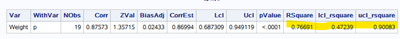## compute 95%CI of r square in proc reg

Hi everyone!

I'm running a weighted linear regression with the following code;

proc reg data=mydata ;
weight variance;
model sur_estim_y= sur_estim_x;
run;

I want to obtain the 95%CI of the Rsquare estimate (or the standard dev of the Rsquare estimate)

In the manual I saw the possibility to compute 95%CI for the parameter estimates but not for the Rsquare.

Does anyone know how to do it?

Thanks a lot

5 REPLIES 5

## Re: compute 95%CI of r square in proc reg

R-squared is simple the square of the correlation coefficient, which is what this paper gets confidence intervals for.

--
Paige Miller

## Re: compute 95%CI of r square in proc reg

Thanks a lot!

I used proc corr and obtained the same Rsquare as from proc reg (corr coefficent **2)

However i'm not sure I how to use standard error from the corr coefficent  to compute 95%CI for the Rsquare estimates

## Re: compute 95%CI of r square in proc reg

You obtain the confidence limits for the correlation coefficient r as shown in the article. Then you square them to get the confidence limits for R-squared.

--
Paige MillerKsharp
Super User

## Re: compute 95%CI of r square in proc reg

Yes. RSquare is just the square of correlation between Y and Yhat(Y predicted value).

You could use fisher options of PROC CORR to get its CI .

``````proc reg data=sashelp.class  ;
weight age;
model weight= height;
output out=want predicted=p;
quit;

ods output FisherPearsonCorr=FisherPearsonCorr;
proc corr data=want outp=outp fisher;
var weight;
with p;
weight age;
run;

data FisherPearsonCorr;
set FisherPearsonCorr;
RSquare=Corr**2;
lcl_rsquare=lcl**2;
ucl_rsquare=ucl**2;
run;

proc print noobs;run;``````Ksharp
Super User

## Re: compute 95%CI of r square in proc reg

Calling @Rick_SAS  @StatDave

Discussion stats
• 5 replies
• 319 views
• 0 likes
• 3 in conversation